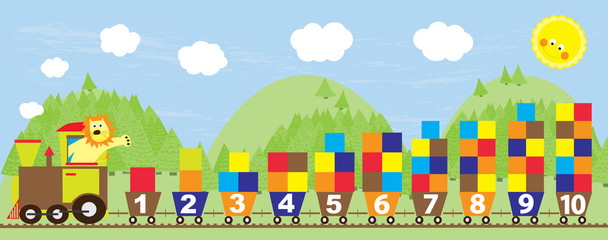A number is a count or measurement. We use numbers to count different quantities, quantify measurements and for making calculations. Numeral is the symbol of number. Each number has an English name.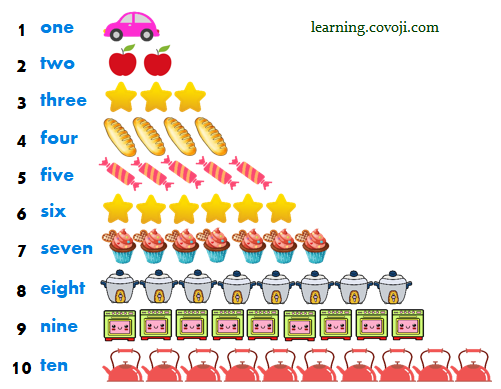##### What do numbers do?

Numbers tell you “how many“. Each number tells you about a different amount.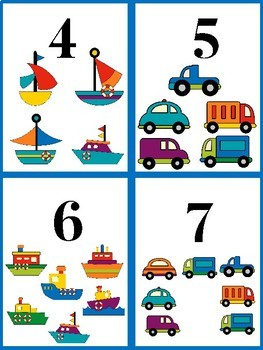Number can use as labels to show which thing is which.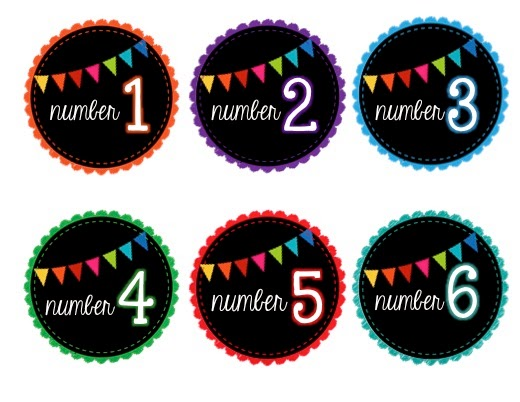Number can use as codes such as telephone numbers and barcode.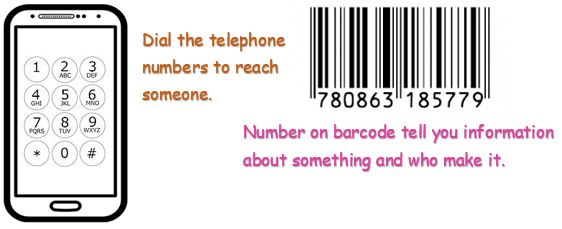Numbers are all around us everyday of life. Counting money, cooking, weighing, price, postcode or telling time.

##### Different types of numbers

There are many types of numbers such as:

• Whole numbers: numbers from O onward. {0, 1, 2, 3, 4……}
• Natural numbers: numbers from 1 onward. Know as positive numbers. {1, 2, 3, 4, 5……}
• Decimal numbers: numbers in the scale of tens, represent numbers smaller than 1. decimal point is a dot used to separate the whole part of a number from fractional part of a number. {0.85, 1.25, 13.86, 100.10……}
• Fraction Numbers: tell us how many parts of a whole we have. { 1/2, 3/8, 2/5……}
• Ordinal Numbers: tell us the position of something in list. {1st, 2nd, 3rd, 4th……}
##### Number Line

Writing down numbers on number line makes it easy for us to see the size of numbers. A number on the left is less than a number on the right. We always move to the right to add, move left to subtract and skip count to multiply.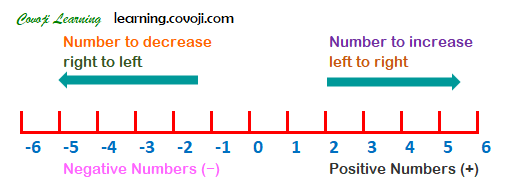Categories: Math Zone